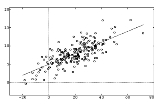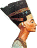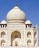xRegression analysis
Posts  1 - 2  of  2abdulmans2002
How do I report regression analysis results?
replied to:  abdulmans2002meyzy
Replied to:  How do I report regression analysis results?
1. See the sig. of your variables. you can see in coefficient table. if you use alpha 0,05, here the interpretation:
a. if the sig. < 0,05 then the variable is significant
b. if the sig. > 0,05 then the variable is not significant
2. See the R square score. the R-square measure gives the proportion of variation in the dependent variable that is explained by the independent variables. if the R square is getting closer to 1,then it shows your models is good.